# lsim

Plot simulated time response of dynamic system to arbitrary inputs; simulated response data

## Syntax

``lsim(sys,u,t)``
``lsim(sys,u,t,x0)``
``lsim(sys,u,t,x0,method)``
``lsim(sys1,sys2,...,sysN,u,t,___)``
``lsim(sys1,LineSpec1,...,sysN,LineSpecN,___)``
``y = lsim(sys,u,t)``
``y = lsim(sys,u,t,x0)``
``y = lsim(sys,u,t,x0,method)``
``[y,tOut,x] = lsim(___)``
``lsim(sys)``

## Description

### Response Plots

example

````lsim(sys,u,t)` plots the simulated time response of the dynamic system model `sys` to the input history (`t`,`u`). The vector `t` specifies the time samples for the simulation. For single-input systems, the input signal `u` is a vector of the same length as `t`. For multi-input systems, `u` is an array with as many rows as there are time samples (`length(t)`) and as many columns as there are inputs to `sys`. ```

example

````lsim(sys,u,t,x0)` further specifies a vector `x0` of initial state values, when `sys` is a state-space model. ```
````lsim(sys,u,t,x0,method)` specifies how `lsim` interpolates the input values between samples, when `sys` is a continuous-time model.```

example

````lsim(sys1,sys2,...,sysN,u,t,___)` simulates the responses of several dynamic system models to the same input history and plots these responses on a single figure. All systems must have the same number of inputs and outputs. You can also use the `x0` and `method` input arguments when computing the responses of multiple models.```

example

````lsim(sys1,LineSpec1,...,sysN,LineSpecN,___)` specifies a color, line style, and marker for each system in the plot. When you need additional plot customization options, use `lsimplot` instead.```

### Response Data

example

````y = lsim(sys,u,t)` returns the system response `y`, sampled at the same times `t` as the input. For single-output systems, `y` is a vector of the same length as `t`. For multi-output systems, `y` is an array having as many rows as there are time samples (`length(t)`) and as many columns as there are outputs in `sys`. This syntax does not generate a plot. ```
````y = lsim(sys,u,t,x0)` further specifies a vector `x0` of initial state values, when `sys` is a state-space model. ```
````y = lsim(sys,u,t,x0,method)` specifies how `lsim` interpolates the input values between samples, when `sys` is a continuous-time model.```

example

````[y,tOut,x] = lsim(___)` returns the state trajectories `x`, when `sys` is a state-space model. `x` is an array with as many rows as there are time samples and as many columns as there are states in `sys`. This syntax also returns the time samples used for the simulation in `tOut`.```

### Linear Simulation Tool

````lsim(sys)` opens the Linear Simulation Tool. For more information about using this tool for linear analysis, see Working with the Linear Simulation Tool.```

## Examples

collapse all

Consider the following transfer function.

`sys = tf(3,[1 2 3])`
```sys = 3 ------------- s^2 + 2 s + 3 Continuous-time transfer function. ```

To compute the response of this system to an arbitrary input signal, provide `lsim` with a vector of the times `t` at which you want to compute the response and a vector `u` containing the corresponding signal values. For instance, plot the system response to a ramping step signal that starts at 0 at time `t = 0`, ramps from 0 at `t = 1` to 1 at `t = 2`, and then holds steady at 1. Define `t` and compute the values of `u`.

```t = 0:0.04:8; % 201 points u = max(0,min(t-1,1));```

Use `lsim` without an output argument to plot the system response to the signal.

```lsim(sys,u,t) grid on```The plot shows the applied input `(u,t)` in gray and the system response in blue.

Use `lsim` with an output argument to obtain the simulated response data.

```y = lsim(sys,u,t); size(y)```
```ans = 1×2 201 1 ```

The vector `y` contains the simulated response at the corresponding times in `t`.

Use `gensig` to create periodic input signals such as sine waves and square waves for use with `lsim`. Simulate the response to a square wave of the following SISO state-space model.

```A = [-3 -1.5; 5 0]; B = [1; 0]; C = [0.5 1.5]; D = 0; sys = ss(A,B,C,D);```

For this example, create a square wave with a period of 10 s and a duration of 20 s.

`[u,t] = gensig("square",10,20);`

`gensig` returns the vector `t` of time steps and the vector `u` containing the corresponding values of the input signal. (If you do not specify a sample time for `t`, then `gensig` generates 64 samples per period.) Use these with `lsim` and plot the system response.

```lsim(sys,u,t) grid on```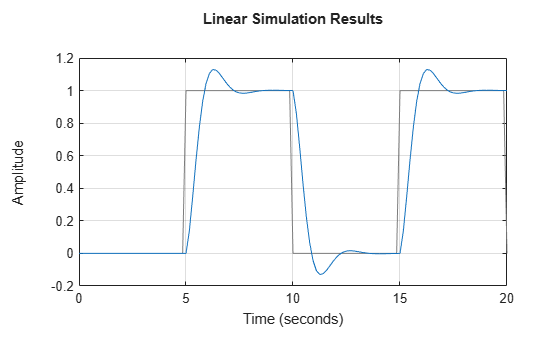The plot shows the applied square wave in gray and the system response in blue. Call `lsim` with an output argument to obtain the response values at each point in `t`.

`[y,~] = lsim(sys,u,t);`

When you simulate the response of a discrete-time system, the time vector `t` must be of the form `Ti:dT:Tf`, where `dT` is the sample time of the model. Simulate the response of the following discrete-time transfer function to a ramp step input.

`sys = tf([0.06 0.05],[1 -1.56 0.67],0.05);`

This transfer function has a sample time of 0.05 s. Use the same sample time to generate the time vector `t` and a ramped step signal `u`.

```t = 0:0.05:4; u = max(0,min(t-1,1));```

Plot the system response.

`lsim(sys,u,t)`To simulate the response of a discrete-time system to a periodic input signal, use the same sample time with `gensig` to generate the input. For instance, simulate the system response to a sine wave with period of 1 s and a duration of 4 s.

`[u,t] = gensig("sine",1,4,0.05);`

Plot the system response.

`lsim(sys,u,t)`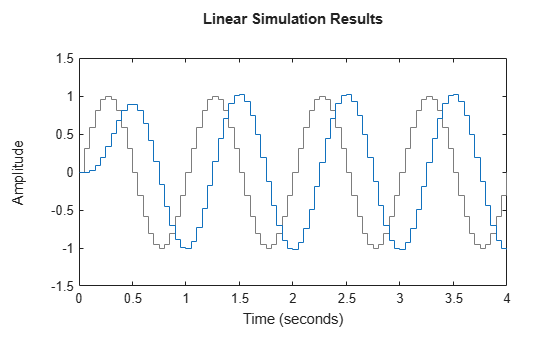`lsim` allows you to plot the simulated responses of multiple dynamic systems on the same axis. For instance, compare the closed-loop response of a system with a PI controller and a PID controller. Create a transfer function of the system and tune the controllers.

```H = tf(4,[1 10 25]); C1 = pidtune(H,'PI'); C2 = pidtune(H,'PID');```

Form the closed-loop systems.

```sys1 = feedback(H*C1,1); sys2 = feedback(H*C2,1);```

Plot the responses of both systems to a square wave with a period of 4 s.

```[u,t] = gensig("square",4,12); lsim(sys1,sys2,u,t) grid on legend("PI","PID")```By default, `lsim` chooses distinct colors for each system that you plot. You can specify colors and line styles using the `LineSpec` input argument.

``` lsim(sys1,"r--",sys2,"b",u,t) grid on legend("PI","PID")```The first `LineSpec` "`r--"` specifies a dashed red line for the response with the PI controller. The second `LineSpec` "`b"` specifies a solid blue line for the response with the PID controller. The legend reflects the specified colors and line styles. For more plot customization options, use `lsimplot`.

In a MIMO system, at each time step `t`, the input `u(t)` is a vector whose length is the number of inputs. To use `lsim`, you specify `u` as a matrix with dimensions `Nt`-by-`Nu`, where `Nu` is the number of system inputs and `Nt` is the length of `t`. In other words, each column of `u` is the input signal applied to the corresponding system input. For instance, to simulate a system with four inputs for 201 time steps, provide `u` as a matrix of four columns and 201 rows, where each row `u(i,:)` is the vector of input values at the `i`th time step; each column `u(:,j)` is the signal applied at the `j`th input.

Similarly, the output `y(t)` computed by `lsim` is a matrix whose columns represent the signal at each system output. When you use `lsim` to plot the simulated response, `lsim` provides separate axes for each output, representing the system response in each output channel to the input `u(t)` applied at all inputs.

Consider the two-input, three-output state-space model with the following state-space matrices.

```A = [-1.5 -0.2 1.0; -0.2 -1.7 0.6; 1.0 0.6 -1.4]; B = [ 1.5 0.6; -1.8 1.0; 0 0 ]; C = [ 0 -0.5 -0.1; 0.35 -0.1 -0.15 0.65 0 0.6]; D = [ 0.5 0; 0.05 0.75 0 0]; sys = ss(A,B,C,D);```

Plot the response of `sys` to a square wave of period 4 s, applied to the first input `sys` and a pulse applied to the second input every 3 s. To do so, create column vectors representing the square wave and the pulsed signal using `gensig`. Then stack the columns into an input matrix. To ensure the two signals have the same number of samples, specify the same end time and sample time.

```Tf = 10; Ts = 0.1; [uSq,t] = gensig("square",4,Tf,Ts); [uP,~] = gensig("pulse",3,Tf,Ts); u = [uSq uP]; lsim(sys,u,t)```Each axis shows the response of one of the three system outputs to the signals `u` applied at all inputs. Each plot also shows all input signals in gray.

By default, `lsim` simulates the model assuming all states are zero at the start of the simulation. When simulating the response of a state-space model, use the optional `x0` input argument to specify nonzero initial state values. Consider the following two-state SISO state-space model.

```A = [-1.5 -3; 3 -1]; B = [1.3; 0]; C = [1.15 2.3]; D = 0; sys = ss(A,B,C,D);```

Suppose that you want to allow the system to evolve from a known set of initial states with no input for 2 s, and then apply a unit step change. Specify the vector `x0` of initial state values, and create the input vector.

```x0 = [-0.2 0.3]; t = 0:0.05:8; u = zeros(length(t),1); u(t>=2) = 1; lsim(sys,u,t,x0) grid on```The first half of the plot shows the free evolution of the system from the initial state values `[-0.2 0.3]`. At `t = 2` there is a step change to the input, and the plot shows the system response to this new signal beginning from the state values at that time.

When you use `lsim` with output arguments, it returns the simulated response data in an array. For a SISO system, the response data is returned as a column vector of the same length as `t`. For instance, extract the response of a SISO system to a square wave. Create the square wave using `gensig`.

```sys = tf([2 5 1],[1 2 3]); [u,t] = gensig("square",4,10,0.05); [y,t] = lsim(sys,u,t); size(y)```
```ans = 1×2 201 1 ```

The vector `y` contains the simulated response at each time step in `t`. (`lsim` returns the time vector `t` as a convenience.)

For a MIMO system, the response data is returned in an array of dimensions N-by-Ny-by-Nu, where Ny and Nu are the number of outputs and inputs of the dynamic system. For instance, consider the following state-space model, representing a three-state system with two inputs and three outputs.

```A = [-1.5 -0.2 1.0; -0.2 -1.7 0.6; 1.0 0.6 -1.4]; B = [ 1.5 0.6; -1.8 1.0; 0 0 ]; C = [ 0 -0.1 -0.2; 0.7 -0.2 -0.3 -0.65 0 -0.6]; D = [ 0.1 0; 0.1 1.5 0 0]; sys = ss(A,B,C,D);```

Extract the responses of the three output channels to the square wave applied at both inputs.

```uM = [u u]; [y,t] = lsim(sys,uM,t); size(y)```
```ans = 1×2 201 3 ```

`y(:,j)` is a column vector containing response at the jth output to the square wave applied to both inputs. That is, `y(i,:)` is a vector of three values, the output values at the ith time step.

Because `sys` is a state-space model, you can extract the time evolution of the state values in response to the input signal.

```[y,t,x] = lsim(sys,uM,t); size(x)```
```ans = 1×2 201 3 ```

Each row of `x` contains the state values `[x1,x2,x3]` at the corresponding time in `t`. In other words, `x(i,:)` is the state vector at the ith time step. Plot the state values.

`plot(t,x)`The example Plot Response of Multiple Systems to Same Input shows how to plot responses of several individual systems on a single axis. When you have multiple dynamic systems arranged in a model array, `lsim` plots all their responses at once.

Create a model array. For this example, use a one-dimensional array of second-order transfer functions having different natural frequencies. First, preallocate memory for the model array. The following command creates a 1-by-5 row of zero-gain SISO transfer functions. The first two dimensions represent the model outputs and inputs. The remaining dimensions are the array dimensions. (For more information about model arrays and how to create them, see Model Arrays.)

`sys = tf(zeros(1,1,1,5));`

Populate the array.

```w0 = 1.5:1:5.5; % natural frequencies zeta = 0.5; % damping constant for i = 1:length(w0) sys(:,:,1,i) = tf(w0(i)^2,[1 2*zeta*w0(i) w0(i)^2]); end```

Plot the responses of all models in the array to a square wave input.

```[u,t] = gensig("square",5,15); lsim(sys,u,t)````lsim` uses the same line style for the responses of all entries in the array. One way to distinguish among entries is to use the `SamplingGrid` property of dynamic system models to associate each entry in the array with the corresponding `w0` value.

`sys.SamplingGrid = struct('frequency',w0);`

Now, when you plot the responses in a MATLAB figure window, you can click a trace to see which frequency value it corresponds to.

Load estimation data to estimate a model.

```load(fullfile(matlabroot,'toolbox','ident','iddemos','data','dcmotordata')); z = iddata(y,u,0.1,'Name','DC-motor');```

`z` is an `iddata` object that stores the one-input two-output estimation data with a sample time of 0.1 s.

Estimate a state-space model of order 4 using estimation data `z`.

`[sys,x0] = n4sid(z,4);`

`sys` is the estimated model and `x0` is the estimated initial states.

Simulate the response of `sys` using the same input data as the one used for estimation and the initial states returned by the estimation command.

`[y,t,x] = lsim(sys,z.InputData,[],x0);`

Here, `y` is the system response, `t` is the time vector used for simulation, and `x` is the state trajectory.

Compare the simulated response `y` to the measured response `z.OutputData` for both outputs.

```subplot(211), plot(t,z.OutputData(:,1),'k',t,y(:,1),'r') legend('Measured','Simulated') subplot(212), plot(t,z.OutputData(:,2),'k',t,y(:,2),'r') legend('Measured','Simulated')```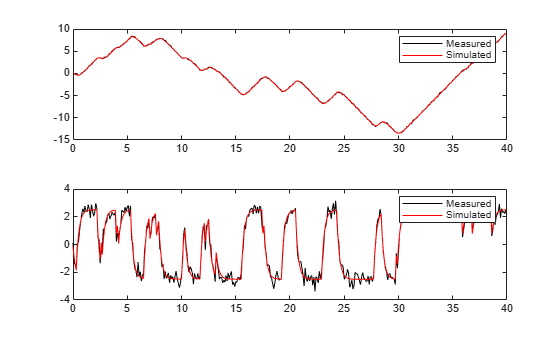The choice of sample time can drastically affect simulation results. To illustrate why, consider the following second-order model.

`$\begin{array}{cc}sys\left(s\right)=\frac{{\omega }^{2}}{{s}^{2}+2s+{\omega }^{2}},& \omega =62.83\end{array}.$`

Simulate the response of this model to a square wave with period 1 s, using a sample time of 0.1 s.

```w2 = 62.83^2; sys = tf(w2,[1 2 w2]); tau = 1; Tf = 5; Ts = 0.1; [u,t] = gensig("square",tau,Tf,Ts); lsim(sys,u,t)```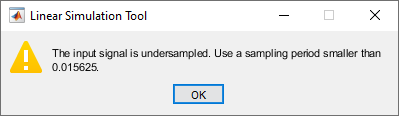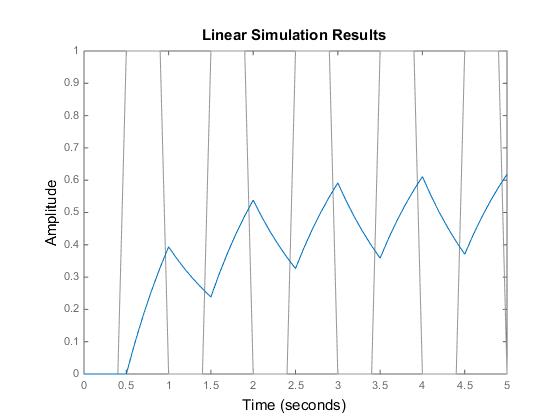`lsim` simulates the model using the specified input signal, but it issues a warning that the input signal is undersampled. `lsim` recommends a sample time that generates at least 64 samples per period of the input `u`. To see why this recommendation matters, simulate `sys` again using a sample time smaller than the recommended maximum.

```figure Ts2 = 0.01; [u2,t2] = gensig("square",tau,Tf,Ts2); lsim(sys,u2,t2)```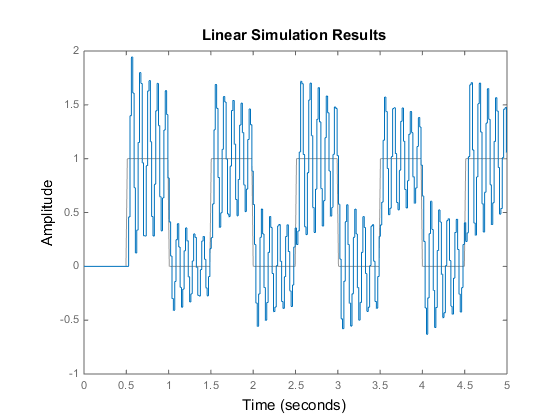This response exhibits strong oscillatory behavior that is hidden in the undersampled version.

## Input Arguments

collapse all

Dynamic system, specified as a SISO or MIMO dynamic system model or array of dynamic system models. Dynamic systems whose responses you can simulate include:

• Continuous-time or discrete-time numeric LTI models, such as `tf`, `zpk`, or `ss` models.

• Generalized or uncertain LTI models such as `genss` or `uss` models. (Using uncertain models requires Robust Control Toolbox™ software.)

• For tunable control design blocks, the function evaluates the model at its current value for both plotting and returning response data.

• For uncertain control design blocks, the function plots the nominal value and random samples of the model. When you use output arguments, the function returns response data for the nominal model only.

• Sparse state-space models such as `sparss` and `mechss` models.

• Identified LTI models, such as `idtf`, `idss`, or `idproc` models. For identified models, you can also use the `sim` (System Identification Toolbox) command, which can compute the standard deviation of the simulated response and state trajectories. `sim` can also simulate all types of models with nonzero initial conditions, and can simulate nonlinear identified models.(Using identified models requires System Identification Toolbox™ software.)

`lsim` does not support frequency-response data models such as `frd`, `genfrd`, or `idfrd` models.

If `sys` is an array of models, the function plots the responses of all models in the array on the same axes. See Response of Systems in Model Array.

Input signal for simulation, specified as a vector for single-input systems, and an array for multi-input systems.

• For single-input systems, `u` is a vector of the same length as `t`.

• For multi-input systems, `u` is an array with as many rows as there are time samples (`length(t)`) and as many columns as there are inputs to `sys`. In other words, each row `u(i,:)` represents the values applied at the inputs of `sys` at time `t(i)`. Each column `u(:,j)` is the signal applied to the `j`th input of `sys`.

Time samples at which to compute the response, specified as a vector of the form `0:dT:Tf`. The `lsim` command interprets `t` as having the units specified in the `TimeUnit` property of the model `sys`.

For continuous-time `sys`, the `lsim` command uses the time step `dT` to discretize the model. If `dT` is too large relative to the system dynamics (undersampling), `lsim` issues a warning recommending a faster sampling time. For further discussion of the impact of sampling time on simulation, see Effect of Sample Time on Simulation.

For discrete-time `sys`, the time step `dT` must equal the sample time of `sys`. Alternatively, you can omit `t` or set it to `[]`. In that case, `lsim` sets `t` to a vector of the same length as `u` that begins at 0 with a time step equal to `sys.Ts`.

Initial state values for simulating a state-space model, specified as a vector having one entry for each state in `sys`. If you omit this argument, then `lsim` sets all states to zero at ```t = 0```.

Discretization method for sampling continuous-time models, specified as one of the following.

• `'zoh'` — Zero-order hold

• `'foh'` — First-order hold

When `sys` is a continuous-time model, `lsim` computes the time response by discretizing the model using a sample time equal to the time step `dT = t(2)-t(1)` of `t`. If you do not specify a discretization method, then `lsim` selects the method automatically based on the smoothness of the signal `u`. For more information about these two discretization methods, see Continuous-Discrete Conversion Methods.

Line style, marker, and color, specified as a string or vector of one, two, or three characters. The characters can appear in any order. You do not need to specify all three characteristics (line style, marker, and color). For example, if you omit the line style and specify the marker, then the plot shows only the marker and no line. For more information about configuring this argument, see the `LineSpec` input argument of the `plot` function.

Example: `'r--'` specifies a red dashed line

Example: `'*b'` specifies blue asterisk markers

Example: `'y'` specifies a yellow line

## Output Arguments

collapse all

Simulated response data, returned as an array.

• For single-input systems, `y` is a column vector of the same length as `t`.

• For multi-output systems, `y` is an array with as many rows as there are time samples (`length(t)`) and as many columns as there are outputs in `sys`. Thus, the jth column of `y`, or `y(:,j)`, contains the response at the jth output to `u` applied at all inputs.

Time vector used for simulation, returned as a column vector. When you specify an input time vector `t` of the form `0:dT:Tf`, then `tOut = t`. If `t` is nearly equisampled, `lsim` adjusts the sample times for simulation and returns the result in `tOut`. For discrete-time `sys`, you can omit `t` or set it to `[]`. In that case, `lsim` sets `t` to a vector of the same length as `u` that begins at 0 with a time step equal to `sys.Ts`, and returns the result in `tOut`.

State trajectories, returned as an array. When `sys` is a state-space model, `x` contains the evolution of the states of `sys` in response to the input. `x` is an array with as many rows as there are time samples `(length(t))` and as many columns as there are states in sys.

## Algorithms

For a discrete-time transfer function,

`$sys\left({z}^{-1}\right)=\frac{{a}_{0}+{a}_{1}{z}^{-1}+\dots +{a}_{n}{z}^{-n}}{1+{b}_{1}{z}^{-1}+\dots +{b}_{n}{z}^{-n}},$`

`lsim` filters the input based on the recursion associated with this transfer function:

`$y\left[k\right]={a}_{0}u\left[k\right]+\dots +{a}_{n}u\left[k-n\right]-{b}_{1}y\left[k-1\right]-\dots -{b}_{n}\left[k-n\right].$`

For discrete-time `zpk` models, `lsim` filters the input through a series of first-order or second-order sections. This approach avoids forming the numerator and denominator polynomials, which can cause numerical instability for higher-order models.

For discrete-time state-space models, `lsim` propagates the discrete-time state-space equations,

`$\begin{array}{c}x\left[n+1\right]=Ax\left[n\right]+Bu\left[n\right],\\ y\left[n\right]=Cx\left[n\right]+Du\left[n\right].\end{array}$`

For continuous-time systems, `lsim` first discretizes the system using `c2d`, and then propagates the resulting discrete-time state-space equations. Unless you specify otherwise with the `method` input argument, `lsim` uses the first-order-hold discretization method when the input signal is smooth, and zero-order hold when the input signal is discontinuous, such as for pulses or square waves. The sample time for discretization is the spacing `dT` between the time samples you supply in `t`.

## Version History

Introduced before R2006a